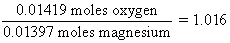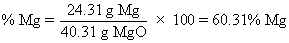# CHEM.1230L: Chemistry I Lab

## Experiment 7: Formula of a Compound, Magnesium & Oxygen

Overview

In this experiment, you precisely weigh a sample of magnesium metal, and then heat the sample in the air. Magnesium metal reacts with the oxygen (O2) of the air to form magnesium oxide. Mg + O2 ®  magnesium oxide

After the magnesium sample has reacted completely, you determine the mass of magnesium oxide product. From these two masses, you calculate the percentage composition of magnesium oxide.

Calculations

Suppose the data below were recorded for this experiment (see Page 33, Section I).

 Mass of magnesium 0.3397 g Mass of crucible, cover, beaker 45.7324 g Mass of crucible, cover, beaker, product ---------- after first heating 46.3105 g after second heating 46.2995 g after third heating (if performed) 46.2991 g

Note that the experiment calls for you to determine in a small beaker the initial mass of magnesium metal taken. The mass of magnesium recorded for Page 33, Section I, part (a) should be only the mass of the magnesium metal itself (not including the mass of the beaker in which the mass determination was made). Your answer should be between 0.3 and 0.4 g (if you have a larger number than this, you are including the mass of the beaker). The masses recorded on Page 33 in Section I, part (c) include the mass of the containers (the mass of the empty containers is recorded in Section I, part (b)). Note that all masses should be determined to four decimal places on the electronic balance: this results in typically 6 significant figures when the masses of containers are included, which provides for the masses of magnesium and magnesium oxide to be determined to four significant figures.

## Page 33, Part II

a. Mass of Product

The mass of product to be used in all subsequent calculations is based on the final mass of the crucible/beaker and contents (after all heatings have been completed) minus the mass of the empty container (before the magnesium was put into it). For the data listed above, calculate the mass of the product, then click here to check your answer.

b. Mass of Oxygen Reacted

Since the product of your reaction should be pure magnesium oxide (containing magnesium and oxygen), and the initial material taken was pure elemental magnesium, the mass of oxygen reacted should just be the difference in these two masses. Using the data above, calculate the mass of oxygen reacted with the sample of magnesium taken, and then click here to check your answer.

c. Moles of magnesium reacted

The number of moles of magnesium reacted is based on the mass of magnesium taken and the molar mass of magnesium (24.31 g per mole of Mg). For the data above, calculate the number of moles of magnesium reacted, then click here to check your answer.

d. Moles of oxygen reacted

The number of moles of oxygen atoms contained in 0.2270 g of oxygen (calculated above) is calculated using the atomic mass of oxygen (16.00 g per mole). Note that oxygen atoms are found in MgO individually….not as O2 molecules. Calculate the number of moles of oxygen atoms contained in 0.2270 g of oxygen, then click here to check your answer.

e. Ratio of moles Mg atoms to moles O atoms

The empirical formula represents the smallest whole number ratio of the types of atoms in a compound. On an experimental bases, we have found that 0.01397 moles of magnesium has combined with 0.01419 moles of oxygen. This means that the number of oxygen atoms that have combined per magnesium atom is given by the ratiof. Empirical formula (small whole numbers)

Since we cannot have "1.016" oxygen atoms, and since there is experimental error in any endeavor, we round off this ratio to the whole number one. This compound contains one oxygen atom for each magnesium atom, and so the empirical formula is simply MgO.

g. Experimental % Mg (by mass) in product

The percent of magnesium (by mass) is based on the mass of magnesium taken and the mass of product which resulted. For the data above, calculate the percent of magnesium (by mass) determined in the experiment, then click here to check your answer.

h. Actual formula of magnesium oxide (based on oxidation numbers)

In the "Nomenclature Workshop" you practiced writing the formulas for compounds, and in many cases you were able to use the location of a given representative element in the Periodic Table to determine the charge of the ion that the element would form in compounds. Since magnesium is a Group 2A element, it forms +2 ions: Mg2+. Since oxygen is a Group 6A element, it forms -2 ions: O2-. So based on these charges, we would predict that the formula of magnesium oxide should be MgO (one +2 ion combined with one -2 ion).

i. Theoretical % Mg by Mass

Based on the predicted formula of magnesium oxide (part h above), we can calculate the "theoretical" percent by mass of magnesium in magnesium oxide.

 Molar mass of MgO = 24.31 g + 16.00 g = 40.31 gj. % error in percent magnesium

This compares your experimental value for the %Mg [part (g) above] with the theoretical % Mg based on the true formula [part (i) above]. If you did your experiment very carefully, this number should be less than 1%. For the data aboveThe minus sign in the error shows that my result was slightly lower than the true value.

## Questions

We can't provide the answers for you, but here are some "helpful hints".

1. When the question says "elemental" oxygen, it refers to the form in which oxygen is found in the atmosphere.
2. When the questions says "elemental" nitrogen, it refers to the form in which nitrogen is found in the atmosphere.
3. This equation is written based on what you did in steps 7 and 8 of the procedure (what did you add to the crucible at this point??) and the information given in the question itself.
4. With this sort of question, consider the most extreme situation. Suppose you had three 1.000 g samples of magnesium. Suppose you heat one sample of magnesium in pure oxygen so that it is completely converted to magnesium oxide (with no worries about magnesium nitride being present. What is the % Mg in this product?? Suppose you heat another sample of magnesium in pure nitrogen to completely convert it to magnesium nitride. What would be the % Mg in this product?? Suppose you heat the third sample in air which is a mixture of magnesium and oxygen, and find that the product is mostly magnesium oxide, but is contaminated with a small amount of magnesium nitride?? How would the % Mg of this mixture of magnesium oxide and magnesium nitride compare with the % Mg in pure MgO and the % Mg in pure Mg3N2??